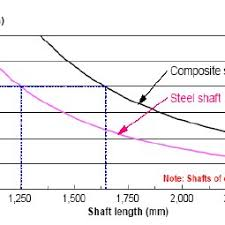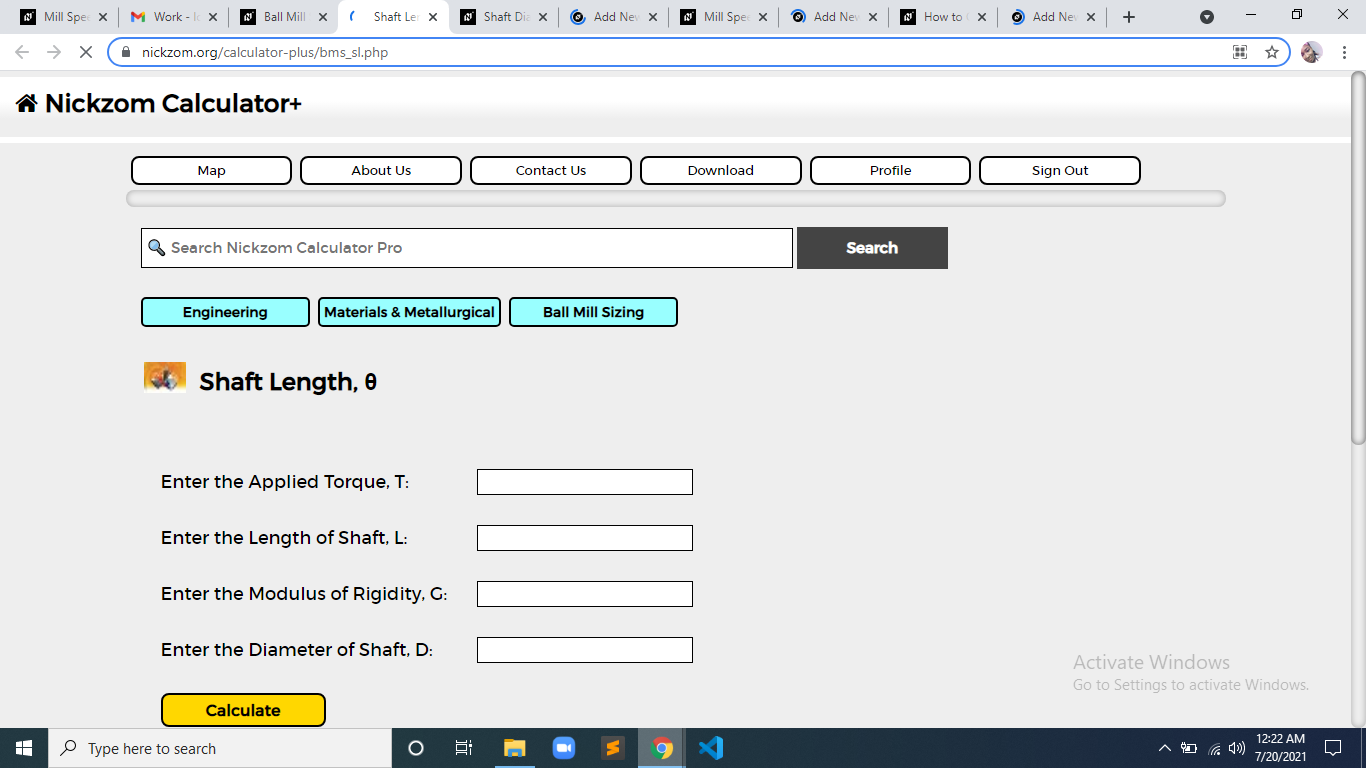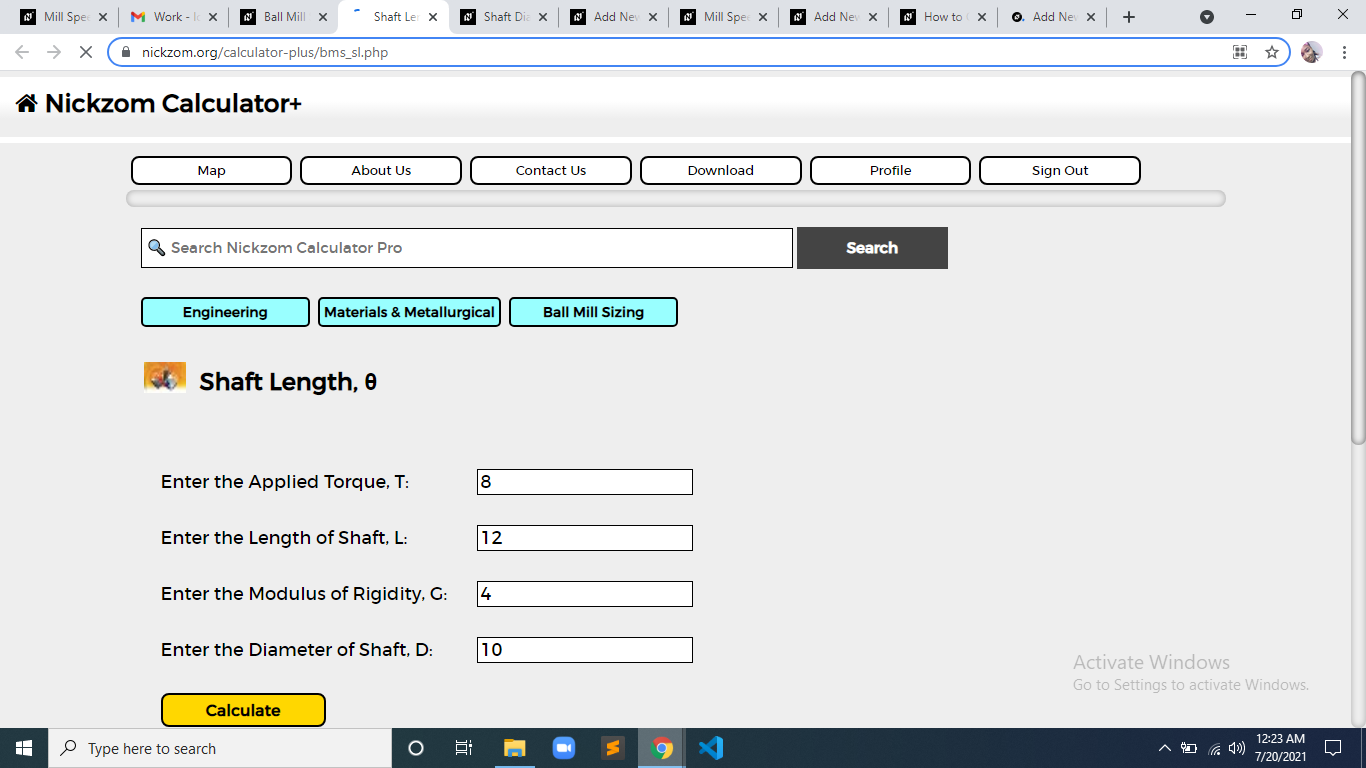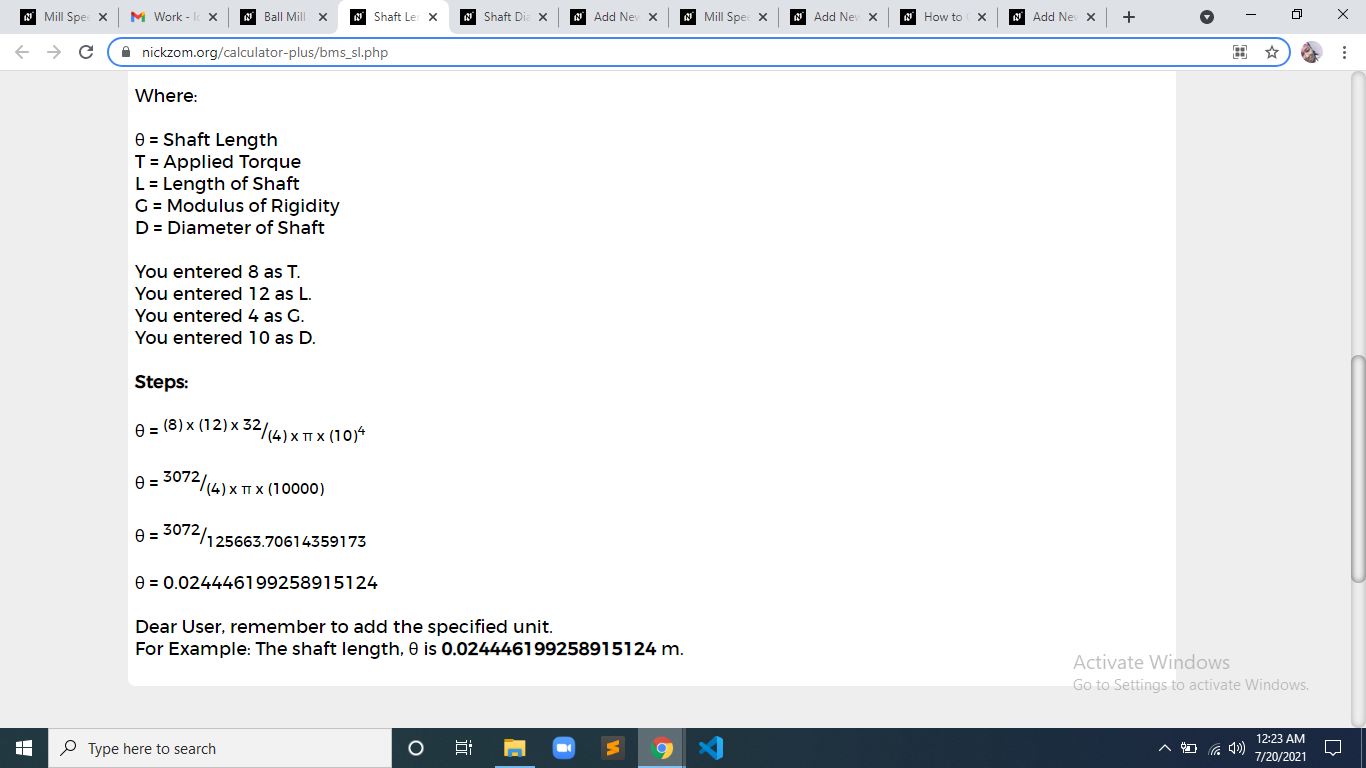# How to Calculate and Solve for Shaft Length | Ball Mill SizingThe image above represents shaft length.

To compute for shaft length, four essential parameters are needed and these parameters are Applied Torque (T), Length of Shaft (L), Modulus of Rigidity (G) and Diameter of Shaft (D).

The formula for calculating shaft length:

θ = T x L x 32/G x π x D4

Where:

θ = Shaft Length
T = Applied Torque
L = Length of Shaft
G = Modulus of Rigidity
D = Diameter of Shaft

Let’s solve an example;
Find the shaft length when the applied torque is 8, the length of shaft is 12, the modulus of rigidity is 4 and the diameter of shaft is 10.

This implies that;

T = Applied Torque = 8
L = Length of Shaft = 12
G = Modulus of Rigidity = 4
D = Diameter of Shaft = 10

θ = T x L x 32/G x π x D4
θ = (8) x (12) x 32/(4) x π x (10)4
θ = 3072/(4) x π x (10000)
θ = 3072/125663.7
θ = 0.024

Therefore, the shaft length is 0.024 m.

Calculating the Applied Torque when the Shaft Length, the Length of Shaft, the Modulus of Rigidity and the Diameter of Shaft is Given.

T = θ x G x π x D4 / L x 32

Where;

T = Applied Torque
θ = Shaft Length
L = Length of Shaft
G = Modulus of Rigidity
D = Diameter of Shaft

Let’s solve an example;
Find the applied torque when the shaft length is 10, the length of shaft is 5, the modulus of rigidity is 4 and the diameter of shaft is 2.

This implies that;

θ = Shaft Length = 10
L = Length of Shaft = 5
G = Modulus of Rigidity = 4
D = Diameter of Shaft = 2

T = θ x G x π x D4 / L x 32
T = 10 x 4 x π x 24 / 5 x 32
T = 40 x π x 16 / 160
T = 2010.6 / 160
T = 12.56

Therefore, the applied torque is 12.56 m.

Calculating the Length of Shaft when the Shaft Length, the Applied Torque, the Modulus of Rigidity and the Diameter of Shaft is Given.

L = θ x G x π x D4 / T x 32

Where;

L = Length of Shaft
θ = Shaft Length
T = Applied Torque
G = Modulus of Rigidity
D = Diameter of Shaft

Let’s solve an example;
Find the length of shaft when the shaft length is 10, the applied torque is 4, the modulus of rigidity is 6 and the diameter of shaft is 2.

This implies that;

θ = Shaft Length = 10
T = Applied Torque = 4
G = Modulus of Rigidity = 6
D = Diameter of Shaft = 2

L = θ x G x π x D4 / T x 32
L = 10 x 6 x π x 24 / 4 x 32
L = 10 x 6 x π x 16 / 4 x 32
L = 3015.9 / 128
L = 23.56

Therefore, the length of shaft is 23.56 m.

Calculating the Modulus of Rigidity when the Shaft Length, the Applied Torque, the Length of Shaft and the Diameter of Shaft is Given.

G = T x L x 32 / θ x π x D4

Where;

G = Modulus of Rigidity
θ = Shaft Length
T = Applied Torque
L = Length of Shaft
D = Diameter of Shaft

Let’s solve an example;
Find the modulus of rigidity when the shaft length is 2, the applied torque is 10, the length of shaft is 6 and the diameter of shaft is 3.

This implies that;

θ = Shaft Length = 2
T = Applied Torque = 10
L = Length of Shaft = 6
D = Diameter of Shaft = 3

G = T x L x 32 / θ x π x D4
G = 10 x 6 x 32 / 2 x π x 34
G = 1920 / 2 x π x 81
G = 1920 / 508.9
G = 3.77

Therefore, the modulus of rigidity is 3.77 m.

Calculating the Diameter of Shaft when the Shaft Length, the Applied Torque, the Length of Shaft and the Modulus of Rigidity is Given.

D = 4√(T x L x 32 / θ x π x G)

Where;

D = Diameter of Shaft
θ = Shaft Length
T = Applied Torque
L = Length of Shaft
G = Modulus of Rigidity

Let’s solve an example;
Find the diameter of shaft when the shaft length is 14, the applied torque is 8, the length of shaft is 4 and the modulus of rigidity is 6.

This implies that;

θ = Shaft Length = 14
T = Applied Torque = 8
L = Length of Shaft = 4
G = Modulus of Rigidity = 6

D = 4√(T x L x 32 / θ x π x G)
D = 4√(8 x 4 x 32 / 14 x π x 6)
D = 4√(1024 / 263.8)
D = 4√(3.88)
D = 7.88

Therefore, the diameter of shaft is 7.88 m.

Nickzom Calculator – The Calculator Encyclopedia is capable of calculating the shaft length.

To get the answer and workings of the shaft length using the Nickzom Calculator – The Calculator Encyclopedia. First, you need to obtain the app.

You can get this app via any of these means:

To get access to the professional version via web, you need to register and subscribe for NGN 2,000 per annum to have utter access to all functionalities.
You can also try the demo version via https://www.nickzom.org/calculator

Apple (Paid) – https://itunes.apple.com/us/app/nickzom-calculator/id1331162702?mt=8
Once, you have obtained the calculator encyclopedia app, proceed to the Calculator Map, then click on Materials and Metallurgical under Engineering.Now, Click on Ball Mill Sizing under Materials and MetallurgicalNow, Click on Shaft Length under Ball Mill SizingThe screenshot below displays the page or activity to enter your values, to get the answer for the shaft length according to the respective parameters which is the Applied Torque (T), Length of Shaft (L), Modulus of Rigidity (G) and Diameter of Shaft (D).Now, enter the value appropriately and accordingly for the parameter as required by the Applied Torque (T) is 8, Length of Shaft (L) is 12, Modulus of Rigidity (G) is 4 and Diameter of Shaft (D) is 14.Finally, Click on CalculateAs you can see from the screenshot above, Nickzom Calculator– The Calculator Encyclopedia solves for the shaft length and presents the formula, workings and steps too.